﻿ Analytical Procedure for Estimating the Gravitational Constant with Nuclear Binding Energy of Stable Atomic Nuclides and Squared Avogadro Number

### Analytical Procedure for Estimating the Gravitational Constant with Nuclear Binding Energy of Stable...

U. V. S. Seshavatharam, S. Lakshminarayana

Frontiers of Astronomy, Astrophysics and Cosmology

## Analytical Procedure for Estimating the Gravitational Constant with Nuclear Binding Energy of Stable Atomic Nuclides and Squared Avogadro Number

U. V. S. Seshavatharam1, S. Lakshminarayana2,1Honorary faculty, I-SERVE, Alakapuri, Hyderabad-35, Telangana, India

2Department of Nuclear Physics, Andhra University, Visakhapatnam-03, AP, India

### Abstract

By considering the strength of Schwarzschild interaction as ‘unity’ and by considering squared Avogadro number as a suitable scaling factor, in the previously published papers the authors made an attempt to understand the basics of nuclear physics and strong interaction. In this paper an attempt is made fit the magnitude of the gravitational constant with nuclear binding energy data of naturally occurring stable atomic nuclides starting from Z=30 to 92. Characteristic binding potential can be taken as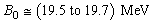. Stable atomic nuclides can be selected in such a way that, ratio of binding energy of the nuclide and characteristic binding potential is close to the proton number of that nuclide. Accuracy of the gravitational constant mainly depends on the selected number of stable atomic nuclides which in turn depends on the accuracy of the assumed binding potential. Very interesting observation is that,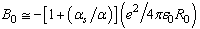where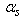is the strong coupling constant,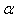is the fine structure ratio and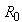is the characteristic nuclear size (1.20 to 1.25) fm. If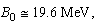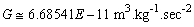and if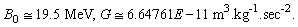• U. V. S. Seshavatharam, S. Lakshminarayana. Analytical Procedure for Estimating the Gravitational Constant with Nuclear Binding Energy of Stable Atomic Nuclides and Squared Avogadro Number. Frontiers of Astronomy, Astrophysics and Cosmology. Vol. 1, No. 2, 2015, pp 105-111. http://pubs.sciepub.com/faac/1/2/4
• Seshavatharam, U. V. S., and S. Lakshminarayana. "Analytical Procedure for Estimating the Gravitational Constant with Nuclear Binding Energy of Stable Atomic Nuclides and Squared Avogadro Number." Frontiers of Astronomy, Astrophysics and Cosmology 1.2 (2015): 105-111.
• Seshavatharam, U. V. S. , & Lakshminarayana, S. (2015). Analytical Procedure for Estimating the Gravitational Constant with Nuclear Binding Energy of Stable Atomic Nuclides and Squared Avogadro Number. Frontiers of Astronomy, Astrophysics and Cosmology, 1(2), 105-111.
• Seshavatharam, U. V. S., and S. Lakshminarayana. "Analytical Procedure for Estimating the Gravitational Constant with Nuclear Binding Energy of Stable Atomic Nuclides and Squared Avogadro Number." Frontiers of Astronomy, Astrophysics and Cosmology 1, no. 2 (2015): 105-111.

 Import into BibTeX Import into EndNote Import into RefMan Import into RefWorks

1
Prev Next

### 1. Introduction

From gravity point of view, so far no model [1-8] succeeded in understanding the link between strongly interacting nucleons and massive celestial objects. By interconnecting the strong coupling constant and gravitational constant via the Schwarzschild interaction and squared Avogadro number, in the previously published papers, the authors reviewed the basics of nuclear structure with reference to up and down quark masses, proton-neutron beta stability, root mean square radius of proton, nuclear binding energy and magic numbers. In this context, readers are strongly encouraged to see the authors published papers [9-17]. In this paper an attempt is made to fit the gravitational constant based on the binding energy of conditionally identified stable atomic nuclides starting from Z=30 to 92. Important point to be noted is that, 19.5 to 19.7 MeV can be considered as the characteristic unified binding energy potential.

### 2. Understanding the Strength of Any Interaction

From the above relations it is reasonable to say that,

1. If it is true that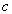and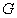are fundamental physical constants, then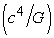can be considered as a fundamental compound constant related to a characteristic limiting force.

2. Black holes are the ultimate state of matter’s geometric structure [18, 19].

3. Magnitude of the operating force at the black hole surface is the order of.

4. Gravitational interaction taking place at black holes can be called as ‘Schwarzschild interaction’.

5. Strength of ‘Schwarzschild interaction’ can be assumed to be unity.

6. Strength of any other interaction can be defined as the ratio of operating force magnitude and the classical or astrophysical force magnitude.

7. If one is willing to represent the magnitude of the operating force as a fraction ofi.e.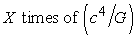, where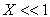, then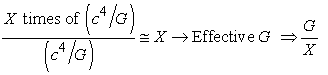(1)

If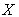is very small,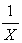becomes very large. In this way,can be called as the strength of interaction. Clearly speaking, strength of any interaction istimes less than the ‘Schwarzschild interaction’ and effectivebecomes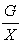.

### 3. Basic Concepts and Relations of Unification Connected with Nuclear Structure

The following concepts and relations can be given a chance in final unification program.

1. Avogadro number is an absolute number [20, 21, 22, 23] and it is having no units like ‘per mole’. Atomic interaction strength is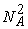times less than the Schwarzschild interaction and hence atomic gravitational constant can be expressed as: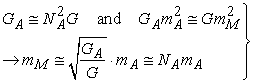(2)

Here,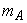is the unified atomic mass unit,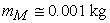is the molar mass unit expressed as ‘one gram’ and hence it is possible to think that,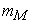constitutes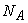number of atoms which in turn constitutesnumber of protons.

2. Similar to the classical force limit, in atomic and nuclear system there exists a characteristic force of magnitude: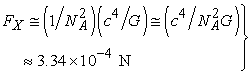(3)

3. In various ‘forms,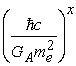where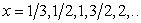seems to play a crucial role in nuclear and atomic physics .

4. Naturally occurring stable mass number connected with proton number can be expressed as follows [24, 25, 26].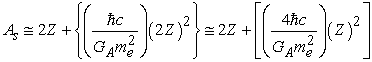(4)

5. For Z>=30, at the stable mass numbers, nuclear binding energy can be expressed as follows.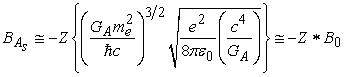(5)

where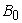can be considered as the characteristic binding energy potential and can be represented as,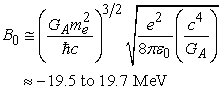(6)

Note that, by considering the semi empirical mass formula [24, 25], at the estimated stable mass numbers of Z>=30, it is possible to show that, ratio of nuclear binding energy and proton number is close to. It is an observed fact.

### 4. To Fit the Gravitational Constant with Nuclear Data

G. Rosi et al say : “There is no definitive relationship between G and the other fundamental constants, and there is no theoretical prediction for its value, against which to test experimental results. Improving the precision with which we know G has not only a pure metrological interest, but is also important because of the key role that G has in theories of gravitation, cosmology, particle physics and astrophysics and in geophysical models”.

In this context, in the previously published papers [12, 13] the authors proposed interesting procedures for estimating the gravitational constant.

From above relations it is possible to show that,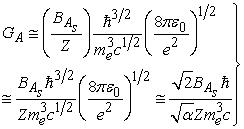(7)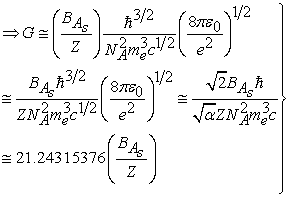(8)

With this simple formula and considering the actual binding energy [24, 25, 26] of naturally occurring stable atomic nuclides starting from Z=30 to Z=92 the mean value of the gravitational constant [27-33] and its standard deviation can be estimated. The important point to be noted is that, to fit the gravitational constant, the authors have chosen the following condition.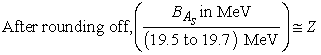(9)

where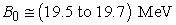is the characteristic binding energy potential that lies close to the value obtained from relation (6). The authors try to fit this energy constant as given below.

Step-1: In a unified approach, it is noticed that,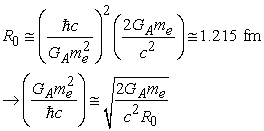(10)

Step-2: Substituting this result in relation (6) it is noticed that,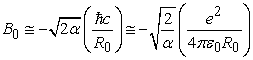(11)

Step-3: From relation (11) it is further noticed that,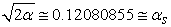(12)

where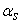represents the currently believed strong coupling constant [20, 23]. Hence relation (11) can be expressed as,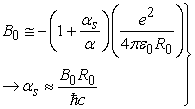(13)

If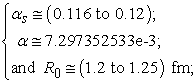By adjusting the value of strong coupling constant in between 0.116 and 0.12 and fixing the characteristic nuclear size with (1.20 to 1.25) fm, value ofcan be shown to lie in between 19.5 MeV and 19.7 MeV. For calculation purpose, in this paper, the authors consider two values ofi.e.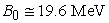and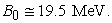Now, by considering the above conditional relation (9), starting from Z=30 to 92, number of stable atomic nuclides can be minimized to 42 for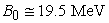and 47 for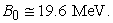After that, by considering relation (8) and by considering the actual binding energies of the conditionally identified stable atomic nuclides, gravitational constant can be estimated for each of the of the atomic nuclide. Average value can be compared with the recommended and other experimental values of the gravitational constant [20,27-33].

### 5. Discussion

From Table 1 it is noticed that, out of 213 stable atomic nuclides , by considering 19.6 MeV as a characteristic binding energy potential, 47 stable atomic nuclides can be identified for fitting gravitational constant. The minimum value of the estimated G is 6.62792E-11 m3.kg-1.sec-2 and maximum value of the estimated G is 6.75292E-11 m3.kg-1.sec-2 Mean of the estimated G for 48 stable atomic nuclides is 6.68541E-11 m3.kg-1.sec-2 and standard deviation is 2.9872E-13 m3.kg-1.sec-2. See Figure 1 and Table 1.

Note that, by considering 19.5 MeV as a characteristic binding energy potential, 42 stable atomic nuclides can be identified for fitting gravitational constant. The minimum value of the estimated G is 6.559016E-11 m3.kg-1.sec-2 and maximum value of the estimated G is 6.73036E-11 m3.kg-1.sec-2 Mean of the estimated G for 42 stable atomic nuclides is 6.64761-11 m3.kg-1.sec-2 and standard deviation is 3.90471E-13 m3.kg-1.sec-2. See Figure 2 and Table 2.Download asVeiw figureFigures index
Figure 1. Estimation of the Gravitational constant with stable atomic nuclides of Z=30 to 92 with 19.6 MeV

#### Table 2. To fit the gravitational constant with stable atomic nuclides with 19.5 MeVDownload asVeiw figureFigures index
Figure 2. Estimation of the Gravitational constant with stable atomic nuclides of Z=30 to 92 with 19.5 MeV

With further research and analysis and by developing the proposed procedure from Z=2 to Z=92, in a suitable manner, accurate value of the gravitational constant can be estimated.

### 6. Conclusion

In general, ‘Unification’ means: a) Understanding the origin of the rest mass of atomic elementary particles. b) Finding and understanding the critical compositeness of the elementary physical constants. c) Minimizing the number of elementary physical constants. d) Merging different branches of physics with possible and suitable physical concepts. In this context, from final unification point of view [9-17], qualitatively and quantitatively, proposed method of estimating the gravitational constant can be recommended for further research and analysis.

### Acknowledgements

Author Seshavatharam U.V.S is indebted to professors K.V. Krishna Murthy, Chairman, Institute of Scientific Research in Vedas (I-SERVE), Hyderabad, India and Shri K.V.R.S. Murthy, former scientist IICT (CSIR), Govt. of India, Director, Research and Development, I-SERVE, for their valuable guidance and great support in developing this subject.

### References

  P. A. M. Dirac, The cosmological constants. Nature, 139, 323, (1937).In article View Article  Witten, Edward. Search for a realistic Kaluza-Klein theory. Nuclear Physics B 186 (3): 412-428. (1981).In article View Article  David Gross, Einstein and the search for Unification. Current science, Vol. 89, No. p 12. (2005).In article  Abdus Salam. Einstein’s Last Dream: The Space -Time Unification of Fundamental Forces, Physics News, 12(2): 36, (1981).In article  Salam A. and Sivaram C. Strong Gravity Approach to QCD and Confinement. Mod. Phys. Lett.,, v. A8(4), 321-326. (1993).In article View Article  Recami E. Elementary Particles as Micro-Universes, and “Strong Black-holes”: A Bi-Scale Approach to Gravitational and Strong Interactions. Preprint NSF-ITP-02-94. posted in the arXives as the e-print physics/0505149, and references therein.In article  Dine, Michael. Supersymmetry and String Theory: Beyond the Standard Model. Cambridge University Press. (2007).In article View Article  Roberto Onofrio. On Weak Interactions as Short-Distance Manifestations of Gravity. Modern Physics Letters A, Vol. 28, No. 7 (2013) 1350022.In article View Article  U. V. S. Seshavatharam, Lakshminarayana S. Understanding Nuclear Stability, Binding Energy and Magic Numbers with Fermi Gas Model. Journal of Applied Physical Science International 4(2), pp.51-59 (2015).In article  U. V. S. Seshavatharam, Lakshminarayana S. Final unification with Schwarzschild’s Interaction. Journal of Applied Physical Science International 3(1): pp.12-22, 2015.In article  U. V. S. Seshavatharam, and S. Lakshminarayana, Gravity Based Integral Charge Quark and Higgs Super Symmetry. Frontiers of Astronomy, Astrophysics and Cosmology, vol. 1, no. 2 (2015): pp.74-89.In article  U. V. S. Seshavatharam and S. Lakshminarayana. On fixing the magnitudes of gravitational constant and strong coupling constant. International Journal of Advanced Astronomy, 3 (1) 17-23, (2015).In article View Article  U. V. S. Seshavatharam and S. Lakshminarayana. On the Plausibility of Final Unification with Avogadro Number. Prespacetime Journal, Volume 5, Issue10, pp. 1028-1041, (2014).In article  U. V. S. Seshavatharam and S. Lakshminarayana. On the Role of Up & Down Quarks in Understanding Nuclear Binding Energy. (Part I) and (Part II)). Prespacetime Journal, Volume 6, Issue 2, pp. 120-142. (2015).In article  U. V. S. Seshavatharam and S. Lakshminarayana. On the role of RMS radius of proton in under-standing nuclear binding energy. Journal of Applied Physical Science International, Vol.: 2, Issue. 3, p84-100. (2015).In article  Seshavatharam, U. V. S. & Lakshminarayana, S., On the Ratio of Nuclear Binding Energy & Protons Kinetic Energy. Prespacetime Journal, Volume 6, Issue 3, pp. 247-255, (2015).In article  U. V. S. Seshavatharam, and S. Lakshminarayana, On the Role of Schwarzschild Interaction in Understanding Strong Interaction and Nuclear Binding Energy. Frontiers of Astronomy, Astrophysics and Cosmology, vol. 1, no. 1: 43-55. (2015).In article  Roger Penrose. Chandrasekhar, Black Holes, and Singularities. J. Astrophys. Astr. (1996) 17, 213-231In article View Article  Subrahmanyan Chandrasekhar. On Stars, Their Evolution and Their Stability',Nobel Prize lecture, December 8, 1983.In article  P.J. Mohr, B.N. Taylor, and D.B. Newell. CODATA Recommended Values of the Fundamental Physical Constants: 2010” by in Rev. Mod. Phys. 84, 1527 (2012) http://pdg.lbl.gov/2014/reviews/rpp2014-rev-phys-constants.pdf.In article  B. Andreas et al. An accurate determination of the Avogadro constant by counting the atoms in a 28Si crystal. Phys. Rev. Let. 106(3):030801 (2011).In article View Article  PubMed  B P Leonard. On the role of the Avogadro constant in redefining SI units for mass and amount of substance. Metrologia 44, 82-86 (2007).In article View Article  K.A. Olive et al. (Particle Data Group), Chin. Phys. C, 38, 090001 (2014).In article View Article  Chowdhury, P.R. et al. Modified Bethe-Weizsacker mass formula with isotonic shift and new driplines. Mod. Phys. Lett. A20 p.1605-1618. (2005).In article View Article  W.D. Myers and W.J. Swiatecki. Table of Nuclear Masses according to the 1994 Thomas-Fermi Model. LBL-36803.(1994).In article  N.Ghahramany et al. New approach to nuclear binding energy in integrated nuclear model. Journal of Theoretical and Applied Physics 6:3 (2012).In article View Article  G. Rosi, F. Sorrentino, L. Cacciapuoti, M. Prevedelli and G. M. Tino1. Precision measurement of the Newtonian gravitational constant using cold atoms. Nature 510, 518-521. (2014).In article View Article  PubMed  L.L. Williams. Analytical Expressions for the Gravitational Constant. (August 2009) http://www.konfluence.org/CalculatingG.pdf.In article  George T Gillies. The Newtonian gravitational constant: recent measurements and related studies. Rep. Prog. Phys. 60 151.In article  J Stuhler et al. MAGIA—using atom interferometry to determine the Newtonian gravitational constant. J. Opt. B: Quantum Semiclass. Opt. 5 (2003) S75-S81.In article View Article  Terry Quinn, Harold Parks, Clive Speake and Richard Davis. An uncertain big G.. Phys.Rev. Lett. 112.068103. (2013).In article  J. B. Fixler; G. T. Foster; J. M. McGuirk; M. A. Kasevich. Atom Interferometer Measurement of the Newtonian Constant of Gravity, Science 315 (5808): 74-77, (2007).In article View Article  PubMed  Jun Luo and Zhong-Kun Hu. Status of measurement of the Newtonian gravitational constant G. Class. Quantum Grav. 17 2351-2363. (2000).In article View Article